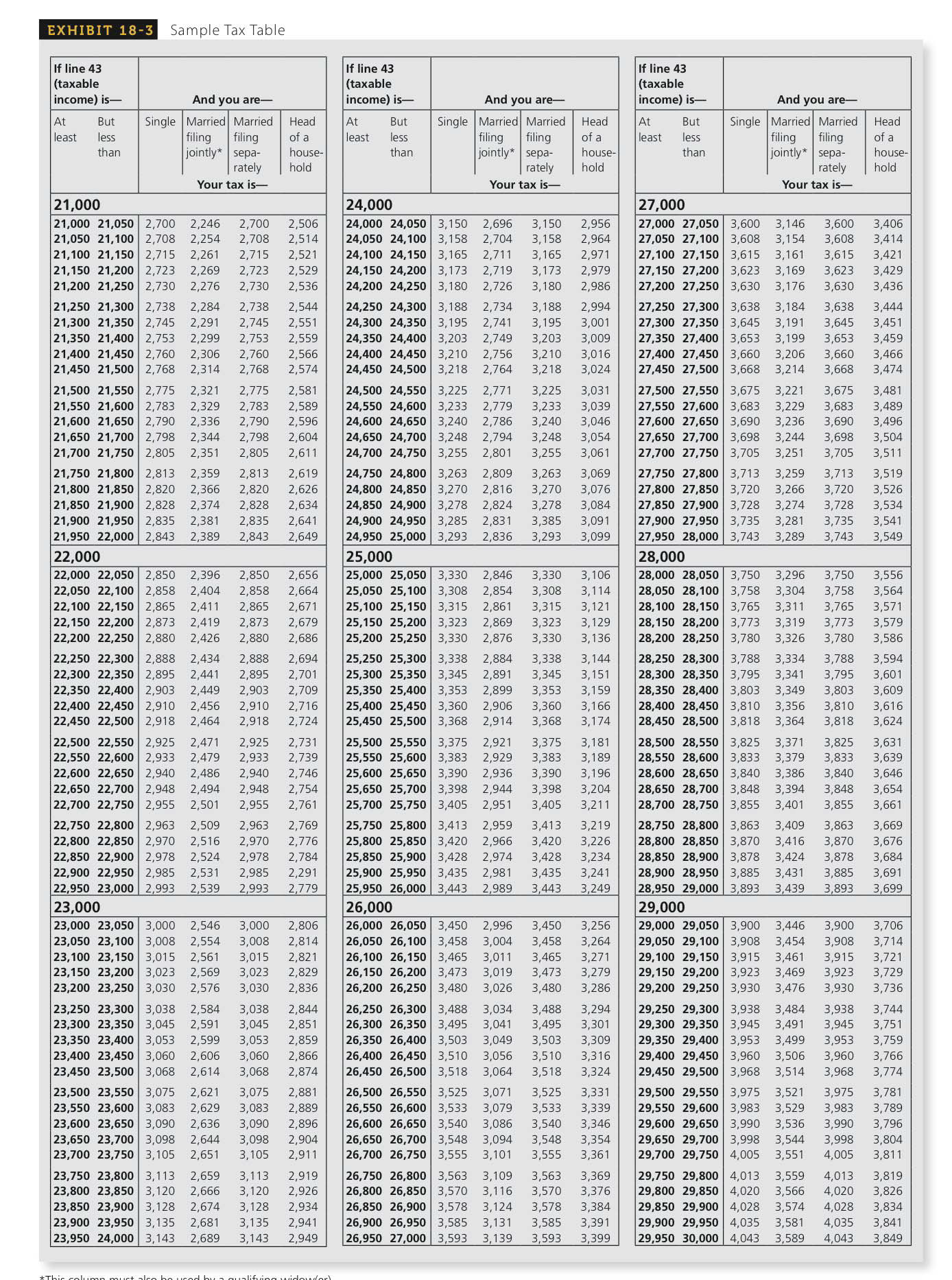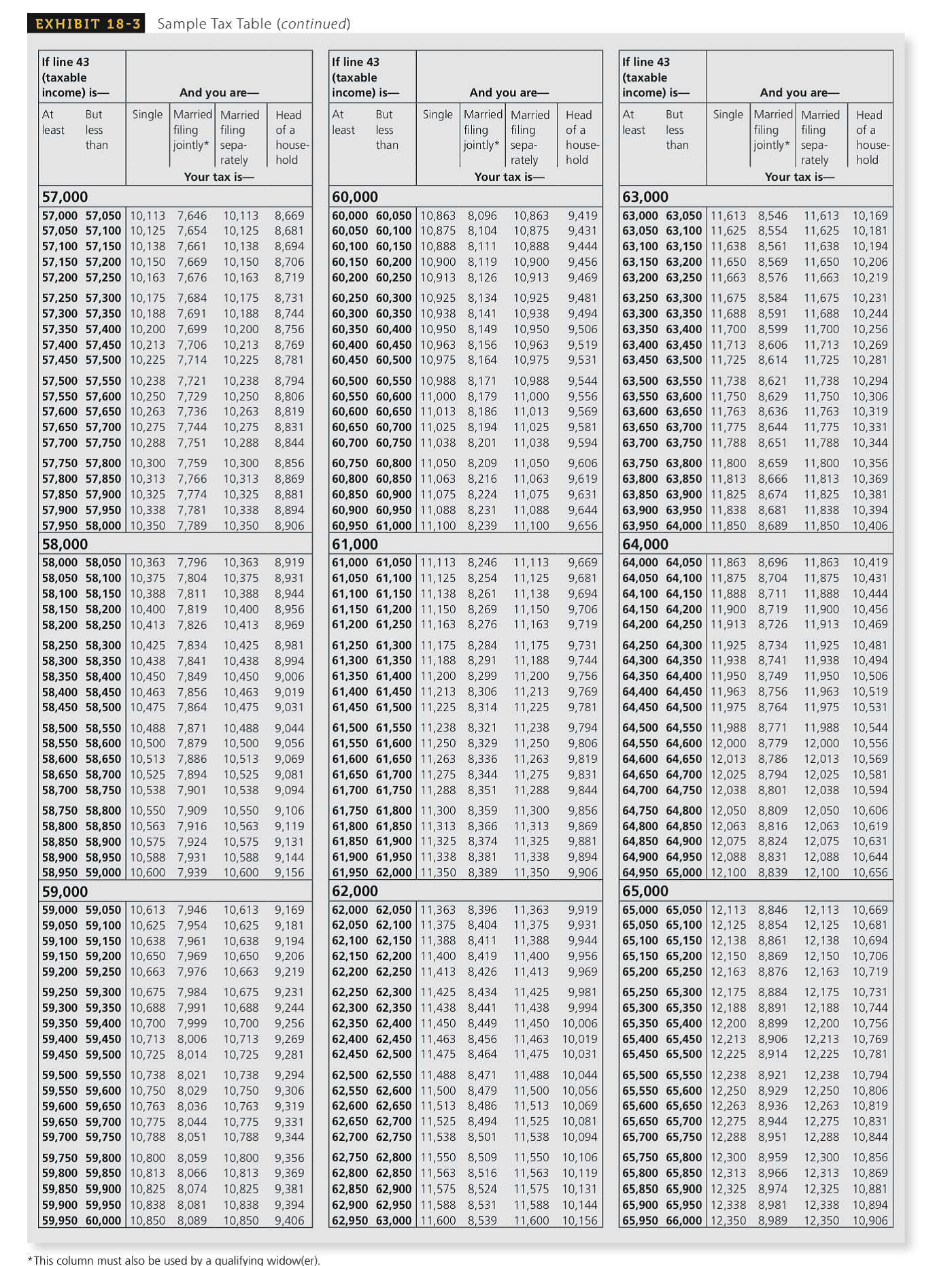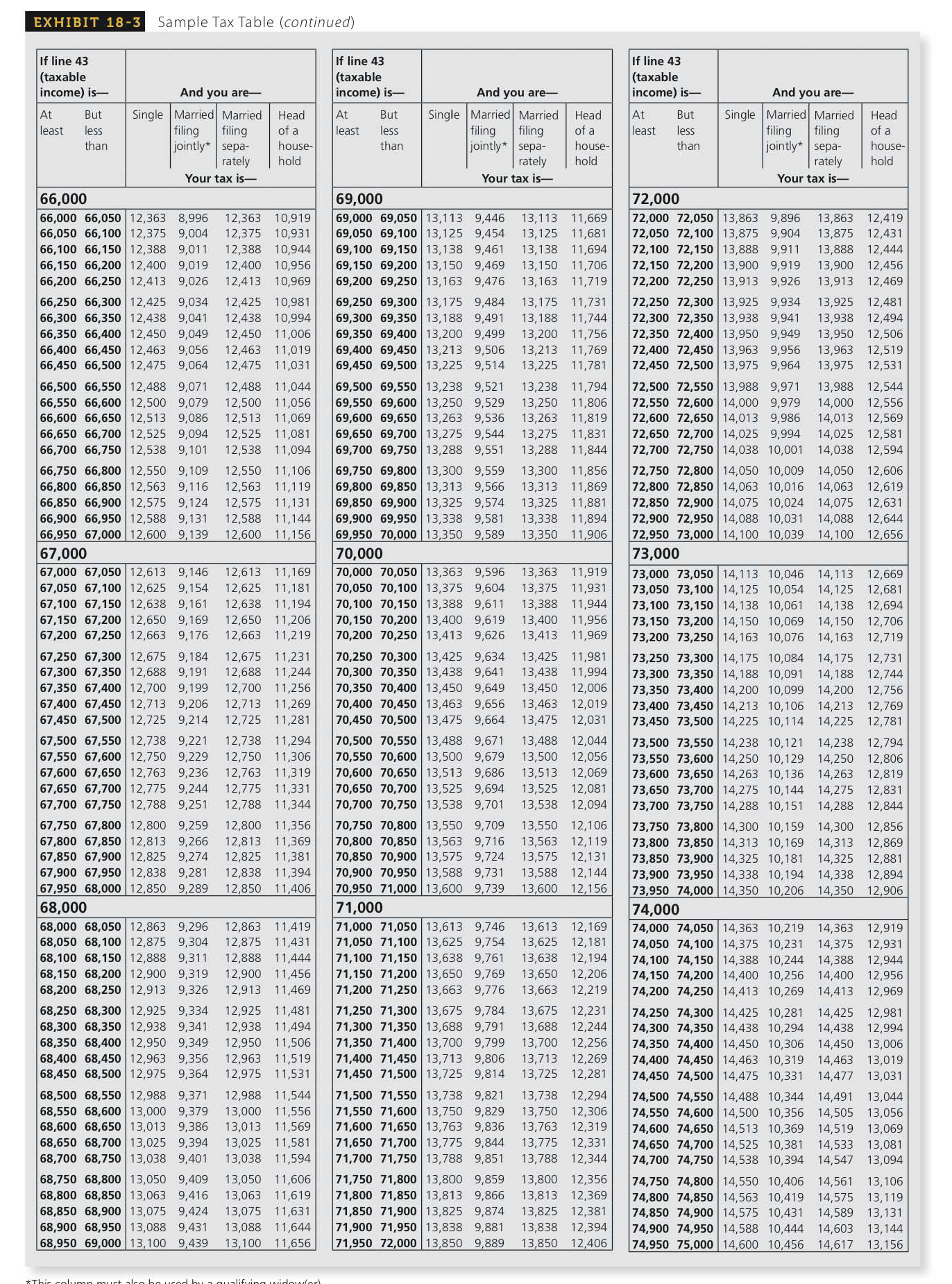Chapter 18.III, Problem 10RE### Contemporary Mathematics for Busin...

8th Edition
Robert Brechner + 1 other
ISBN: 9781305585447

#### Solutions

Chapter
Section### Contemporary Mathematics for Busin...

8th Edition
Robert Brechner + 1 other
ISBN: 9781305585447
Textbook Problem

# Use the Tax Table, Exhibit 18-3, to calculate the tax liability for the following taxpayers earning under $100,000.Name Filing Status Taxable Income lax Liability 10. Julian Single$62,340 $11,438 To determine To calculate: The tax liability for the tax payer Julian whose taxable income is 62,340. Explanation Given Information: The Person Julian is single and has the taxable income of$62,340.

Calculation:

Taxable income is defined as the income to which tax rates are functional in order to discover the total amount of tax payable for the year. Tax table is defined as the table which is used to find the income tax for singles whose earnings are less than \$100,000.

The followings steps are used to calculate

Step 1: Open the tax table EXHIBIT 18-3 and it is needed to find the line which contains the amount of taxable income...

### Still sussing out bartleby?

Check out a sample textbook solution.

See a sample solution

#### The Solution to Your Study Problems

Bartleby provides explanations to thousands of textbook problems written by our experts, many with advanced degrees!

Get Started

#### Find more solutions based on key concepts